Next: 6.4.3.1 Shooting with Reduced Up: 6.4.3 Shooting Method Previous: 6.4.3 Shooting Method

### Single Shooting

Single shooting is based on the principle of superposition valid for linear ODEs,e i.e., any linear combination v(z) =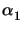v1(z) +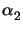v2(z) of two individual solutions v1(z) and v2(z) satisfying the ODE v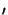(z) =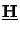(z)v(z) is again a solution of it. The basic theory of ODEs states that an N-dimensional ODE system has exactly N linearly independent solution vectors vi(z) with i = 1,..., N [200, pp. 85-93]. The BCs can be chosen arbitrarily provided that the vectors vi(z) are linearly independent and thus span the whole N-dimensional vector space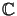N. By taking the unit vectors ei, i.e., [ei]j =, as initial values at one boundary point, e.g., at z = 0, the obtained solutions vi(z) are called fundamental solutions,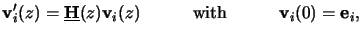(6.36)

that are arranged in the N x N-dimensional fundamental solution matrix V(z). The solution u(z) of any first-order, N-dimensional BVP can be represented by a specific superposition of the fundamental solutions, i.e.,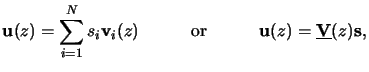(6.37)

with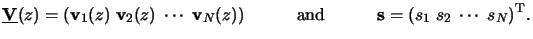(6.38)

The coefficients si have to be chosen so that the imposed BCs are satisfied, i.e., in our case they take the form (cf. (6.51))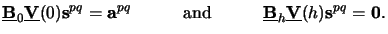(6.39)

These two equations build up a linear algebraic system. Due to the multiple excitation vectors apq we obtain an algebraic equation with Ns right-hand sides and thus Ns coefficient vectors spq, i.e.,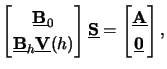(6.40)

whereby all the Ns vectors apq and spq are grouped together in the two matrices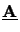and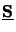, respectively (cf. (6.54)). Note that(0) =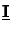has already been inserted for the fundamental solutions at z = 0. (6.59) can be efficiently solved with LU-factorization [202, pp. 94-104] to obtain simultaneously all the coefficients spq looked for. With them the solution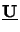(z) can directly be calculated from (6.56) if the complete fundamental solution matrix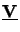(z) is stored for all interesting vertical positions. Otherwise the initial values at z = 0 are simply given by(0) =since(0) =and the IVP thus obtained can easily be integrated. An algorithm implementing the above procedure is described in Table 6.2. As already mentioned this algorithm suffers from potential instabilities [200, pp. 137-143] and has thus to be further refined for our problem.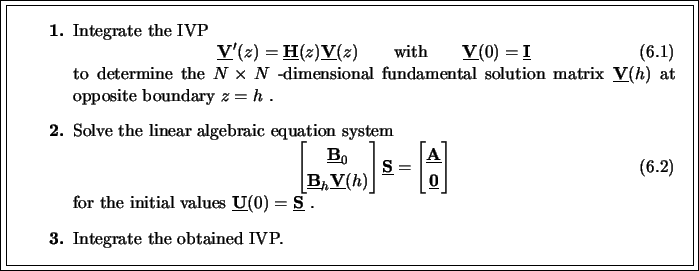#### Footnotes

... ODEs,e
This does not mean that shooting is restricted to linear ODEs. In the linear case, however, the theory can be presented in a very compact and elegant way, whereas in the nonlinear case the treatment is more complicated and additional steps are required. Since our problem is linear we restrict the discussion to this simpler situation.Next: 6.4.3.1 Shooting with Reduced Up: 6.4.3 Shooting Method Previous: 6.4.3 Shooting Method
Heinrich Kirchauer, Institute for Microelectronics, TU Vienna
1998-04-17